# Brick weight

Half the weight of a brick plus 20 pounds is equal to 1/3 the weight of the brick plus 30 pounds. How much does the brick weigh?

x =  60 lb

### Step-by-step explanation:

x/2 + 20 = x/3 + 30

x = 60

Our simple equation calculator calculates it.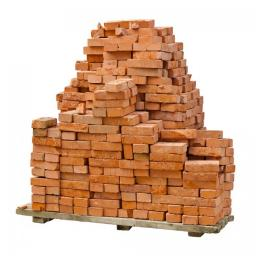Did you find an error or inaccuracy? Feel free to write us. Thank you!Tips to related online calculators
Need help to calculate sum, simplify or multiply fractions? Try our fraction calculator.
Do you have a linear equation or system of equations and looking for its solution? Or do you have a quadratic equation?

## Related math problems and questions:

• Brick weightThe brick weighs 3 kg and a half-brick. How much does one brick weigh?
• Brick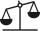Isosceles scale has on one side all brick and second weight 1 kg and 1/4 of brick. The balance is in equilibrium. What is the weight of a brick?
• Two bricks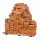One brick weighs 1kg and half a brick, how many weigh two bricks?
• Reducing scale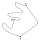I am sizing some landscaping and I measured everything for a 20 to 1 scale. I realized when I was done that it was at 30 to 1. How can I convert what I already have to 30 to 1. (Example, grass area is 22,871.6' at 20 to 1, how do you figure it out to be 3
• PoundsThree pounds subtract 1/3 of a pound.
• Reciprocals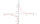Which among the given reciprocal is correct a. 3/15x1/3= 1 b. 3/20x20/3=1 c. 7/14x7/7=1 d. 34/3x34/34=1
• Empty aquariumHow much does an empty aquarium weigh with dimensions: length = 40 cm, width = 30 cm, height = 20 cm, if 1 dm2 of glass weighs 300 g? Calculate its weight in kilograms.
• Emily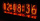Emily had 20 minutes to do a three-problem quiz. She spent 11 3/4 minutes on question A and 5 1/2 minutes on question B. How much time did she have left for question C?
• 30 eggs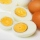There are 30 eggs in a tray. If 1/2 of the tray used 1/5 of it cooked,1/3 kept the refrigerator, how many eggs were left?
• Fractions and mixed numerals(a) Convert the following mixed numbers to improper fractions. i. 3 5/8 ii. 7 7/6 (b) Convert the following improper fraction to a mixed number. i. 13/4 ii. 78/5 (c) Simplify these fractions to their lowest terms. i. 36/42 ii. 27/45 2. evaluate the follow
• Weigh in totalI put 3/5 kg of grapes into a box which is 1/4kg in weight. How many kilograms do the grapes and the box weigh in total?
• Guess a fractionTom was asked to guess a fraction. The sum of 1/2 the numerator and 1/3 of its denominator is 30. If Tom subtracts 36 from its denominator, the fraction becomes 1/3. What is the fraction that Tom was asked to guess? (Leave your answer in simplest form)
• FractionsSort fractions z1 = (6)/(11); z2 = (10)/(21); z3 = (19)/(22) by its size. Result write as three serial numbers 1,2,3.
• Simplest form of a fractionWhich one of the following fraction after reducing in simplest form is not equal to 3/2? a) 15/20 b) 12/8 c) 27/18 d) 6/4
• Water 31Richard takes 3 1/6 liters of water before noon and 2 3/5 liters of water afternoon. How many liters of water does Richard consume a day?
• Lengths of the poolMiguel swam 6 lengths of the pool. Mat swam 3 times as far as Miguel. Lionel swam 1/3 as far as Miguel. How many lengths did Mat swim?
• Dog food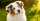A whole bag of dog food weighs 5 1/2 pounds. How much dog food do you have if there are 3 3/4 bags in the cupboard?